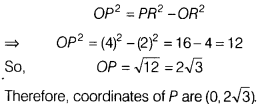# PQR is an equilateral triangle with coordinates of Q and R as (-2,0)and(2,0) respectively

In the given figure, PQR is an equilateral triangle with coordinates of Q and R as (-2,0) and (2,0) respectively. Find the coordinates of the vertex P.from the given figure
QR=2 + 2 = 4
So, PQ=QR = RP = 4
Now, QR = 4
[∵ PQR is an equilateral triangle]
In right angled ∆OPR,